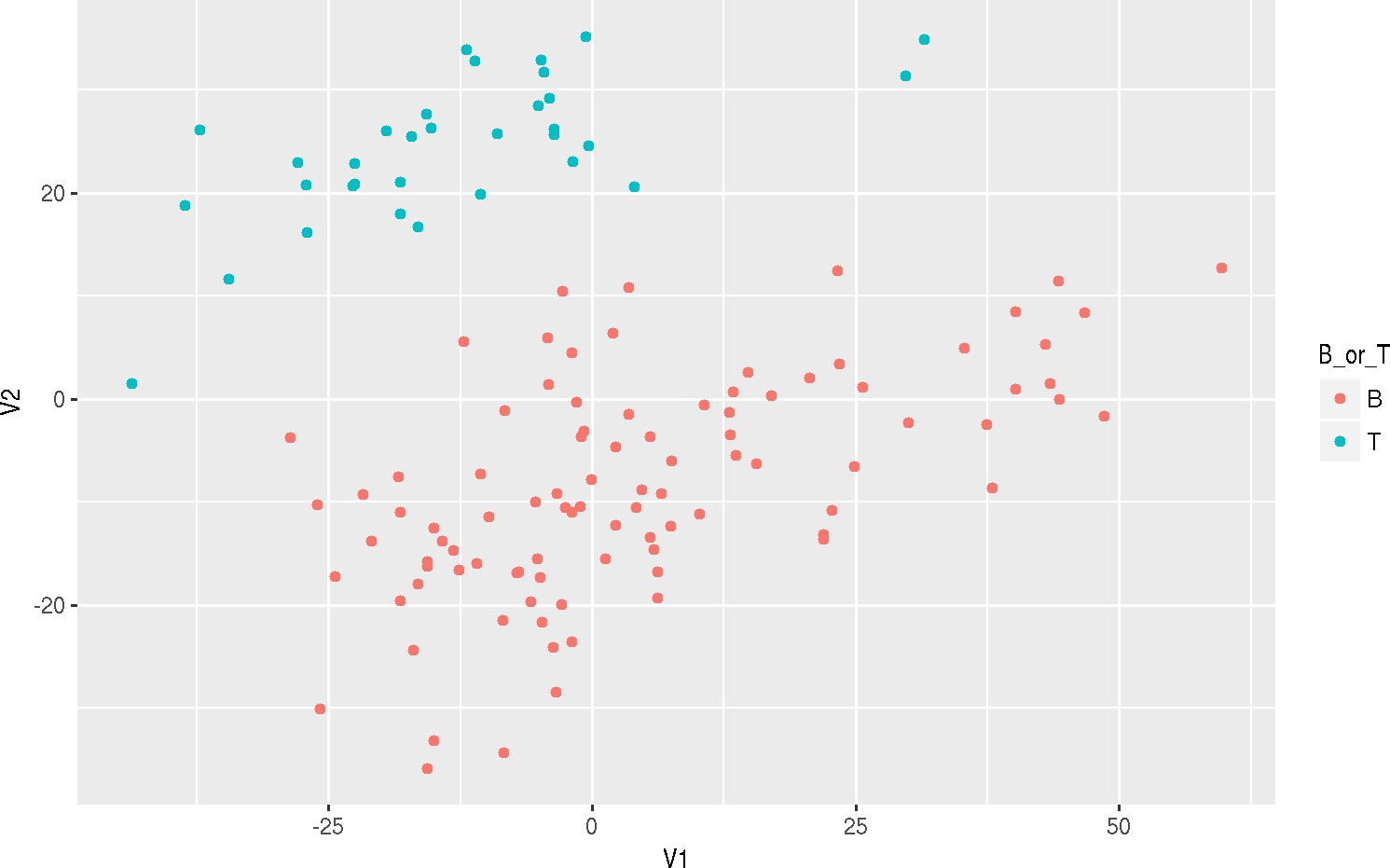# 1 Exploration, univariate, and bivariate statistics and visualization

Input clean data, with Sex and Year as factors.

path <- file.choose()    # look for BRFSS-subset.csv
stopifnot(file.exists(path))
library(tidyverse)
col_types <- cols(
Age = col_integer(),
Weight = col_double(),
Sex = col_factor(c("Female", "Male")),
Height = col_double(),
Year = col_factor(c("1990", "2010"))
)
brfss <- read_csv(path, col_types = col_types)
brfss
## # A tibble: 20,000 x 5
##      Age   Weight    Sex Height   Year
##    <int>    <dbl> <fctr>  <dbl> <fctr>
##  1    31 48.98798 Female 157.48   1990
##  2    57 81.64663 Female 157.48   1990
##  3    43 80.28585   Male 177.80   1990
##  4    72 70.30682   Male 170.18   1990
##  5    31 49.89516 Female 154.94   1990
##  6    58 54.43108 Female 154.94   1990
##  7    45 69.85323   Male 172.72   1990
##  8    37 68.03886   Male 180.34   1990
##  9    33 65.77089 Female 170.18   1990
## 10    75 70.76041 Female 152.40   1990
## # ... with 19,990 more rows

## 1.1 Univariate: t.test() for Weight in 1990 vs. 2010 Females

Filter the data to include females only, and use base plot() function and the formula interface to visualize the relationship between Weight and Year.

brfss %>% filter(Sex %in% "Female") %>% plot(Weight ~ Year, data = .)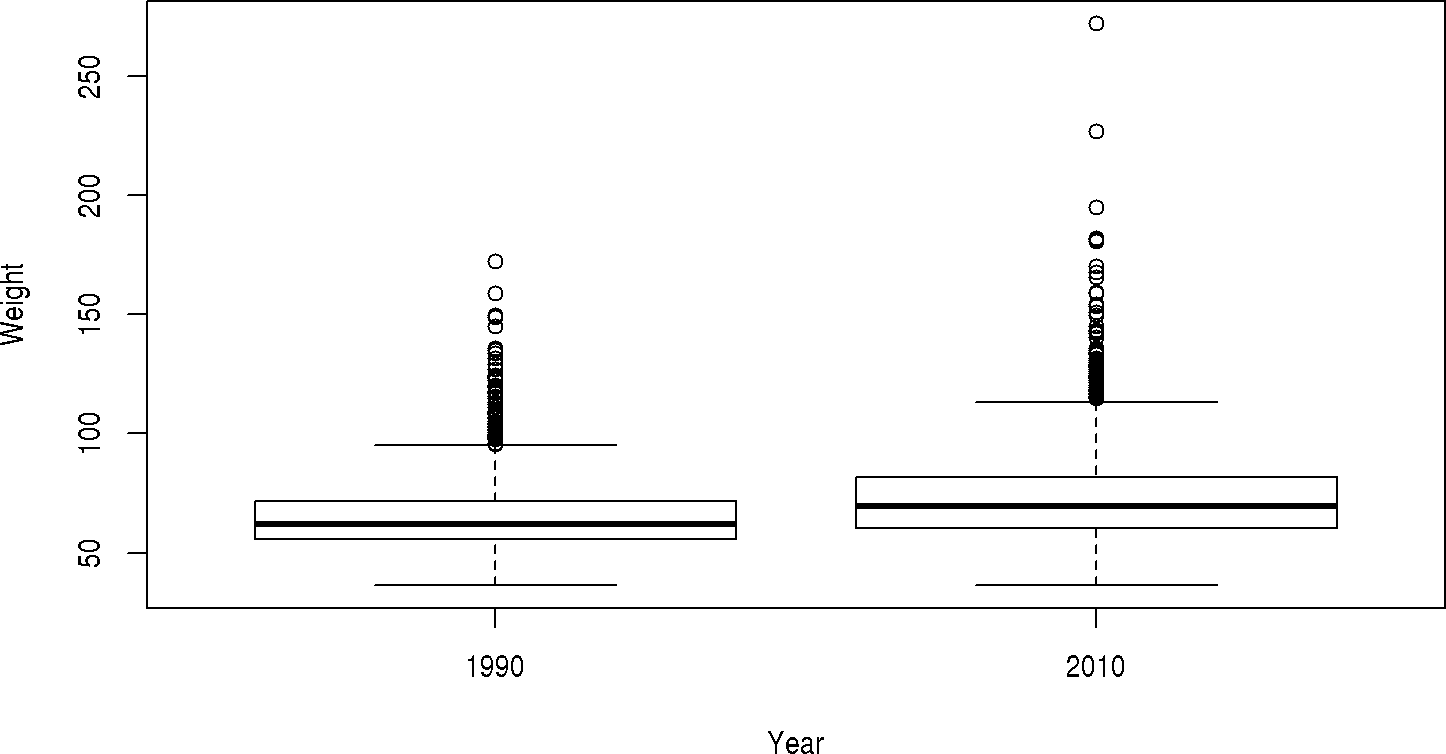Use a t.test() to test the hypothesis that female weight is the same in 2010 as in 1990

brfss %>% filter(Sex %in% "Female") %>% t.test(Weight ~ Year, data = .)
##
##  Welch Two Sample t-test
##
## data:  Weight by Year
## t = -27.133, df = 11079, p-value < 2.2e-16
## alternative hypothesis: true difference in means is not equal to 0
## 95 percent confidence interval:
##  -8.723607 -7.548102
## sample estimates:
## mean in group 1990 mean in group 2010
##           64.81838           72.95424

## 1.2 Bivariate: Weight and height in 2010 Males

Filter the data to contain 2010 Males. Use plot() to visualize the relationship, and lm() to model it.

male2010 <- brfss %>% filter(Year %in% "2010", Sex %in% "Male")
male2010 %>% plot( Weight ~ Height, data = .)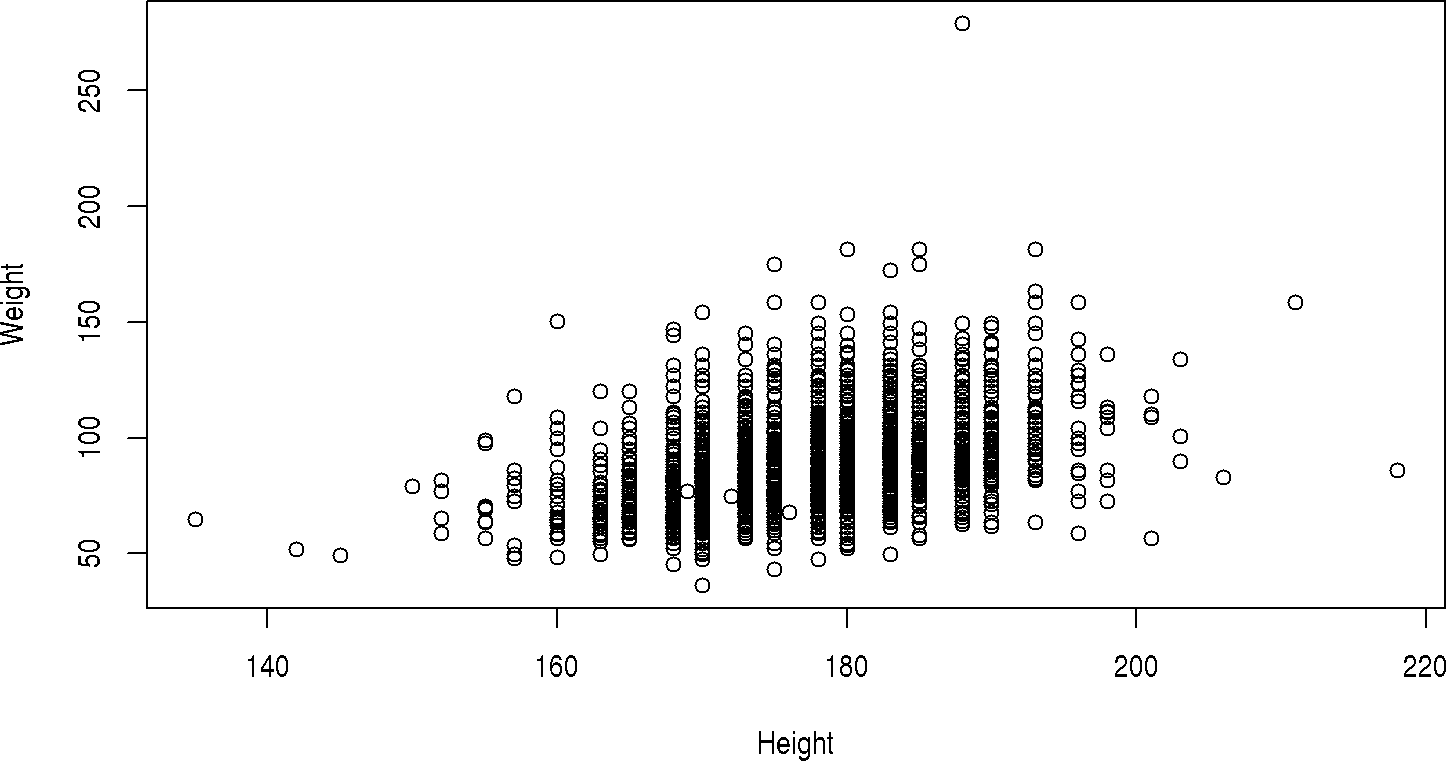fit <- male2010 %>% lm( Weight ~ Height, data = .)
fit
##
## Call:
## lm(formula = Weight ~ Height, data = .)
##
## Coefficients:
## (Intercept)       Height
##    -86.8747       0.9873
summary(fit)
##
## Call:
## lm(formula = Weight ~ Height, data = .)
##
## Residuals:
##     Min      1Q  Median      3Q     Max
## -54.867 -11.349  -2.677   8.263 180.227
##
## Coefficients:
##              Estimate Std. Error t value Pr(>|t|)
## (Intercept) -86.87470    6.67835  -13.01   <2e-16 ***
## Height        0.98727    0.03748   26.34   <2e-16 ***
## ---
## Signif. codes:  0 '***' 0.001 '**' 0.01 '*' 0.05 '.' 0.1 ' ' 1
##
## Residual standard error: 16.88 on 3617 degrees of freedom
##   (60 observations deleted due to missingness)
## Multiple R-squared:  0.1609, Adjusted R-squared:  0.1607
## F-statistic: 693.8 on 1 and 3617 DF,  p-value: < 2.2e-16

Multiple regression: Weight and Height, accounting for difference between years

male <- brfss %>% filter(Sex %in% "Male")
male %>% lm(Weight ~ Year + Height, data = .) %>% summary()
##
## Call:
## lm(formula = Weight ~ Year + Height, data = .)
##
## Residuals:
##     Min      1Q  Median      3Q     Max
## -53.037  -9.032  -1.546   6.846 181.022
##
## Coefficients:
##              Estimate Std. Error t value Pr(>|t|)
## (Intercept) -80.56568    3.88047  -20.76   <2e-16 ***
## Year2010      7.86690    0.32684   24.07   <2e-16 ***
## Height        0.90764    0.02174   41.75   <2e-16 ***
## ---
## Signif. codes:  0 '***' 0.001 '**' 0.01 '*' 0.05 '.' 0.1 ' ' 1
##
## Residual standard error: 14.44 on 7854 degrees of freedom
##   (104 observations deleted due to missingness)
## Multiple R-squared:  0.2262, Adjusted R-squared:  0.2261
## F-statistic:  1148 on 2 and 7854 DF,  p-value: < 2.2e-16

Is there an interaction between Year and Height?

male %>% lm(Weight ~ Year * Height, data = .) %>% summary()
##
## Call:
## lm(formula = Weight ~ Year * Height, data = .)
##
## Residuals:
##     Min      1Q  Median      3Q     Max
## -54.867  -9.080  -1.731   6.796 180.227
##
## Coefficients:
##                  Estimate Std. Error t value Pr(>|t|)
## (Intercept)     -68.49361    5.27146 -12.993  < 2e-16 ***
## Year2010        -18.38109    7.77065  -2.365 0.018032 *
## Height            0.83990    0.02955  28.421  < 2e-16 ***
## Year2010:Height   0.14737    0.04359   3.381 0.000726 ***
## ---
## Signif. codes:  0 '***' 0.001 '**' 0.01 '*' 0.05 '.' 0.1 ' ' 1
##
## Residual standard error: 14.43 on 7853 degrees of freedom
##   (104 observations deleted due to missingness)
## Multiple R-squared:  0.2274, Adjusted R-squared:  0.2271
## F-statistic: 770.3 on 3 and 7853 DF,  p-value: < 2.2e-16

Check out other things to do with fitted model:

• broom::tidy(): P-value, etc., as data.frame
• broom::augment(): fitted values, residuals, etc

library(broom)
male %>% lm(Weight ~ Year + Height, data = .) %>%
augment() %>% as.tibble()
## # A tibble: 7,857 x 11
##    .rownames   Weight   Year Height  .fitted   .se.fit     .resid
##        <chr>    <dbl> <fctr>  <dbl>    <dbl>     <dbl>      <dbl>
##  1         1 80.28585   1990 177.80 80.81238 0.2219842  -0.526530
##  2         2 70.30682   1990 170.18 73.89618 0.2823537  -3.589360
##  3         3 69.85323   1990 172.72 76.20158 0.2519471  -6.348353
##  4         4 68.03886   1990 180.34 83.11778 0.2265455 -15.078925
##  5         5 88.45051   1990 180.34 83.11778 0.2265455   5.332732
##  6         6 81.64663   1990 182.88 85.42318 0.2438568  -3.776555
##  7         7 92.98644   1990 182.88 85.42318 0.2438568   7.563255
##  8         8 97.52236   1990 193.04 94.64478 0.3911687   2.877575
##  9         9 78.01789   1990 170.18 73.89618 0.2823537   4.121711
## 10        10 77.11070   1990 175.26 78.50698 0.2309296  -1.396276
## # ... with 7,847 more rows, and 4 more variables: .hat <dbl>, .sigma <dbl>,
## #   .cooksd <dbl>, .std.resid <dbl>

## 1.3 Visualization: ggplot2

ggplot: “Grammar of Graphics”

• data: ggplot2()
• aesthetics: aes(), ‘x’ and ‘y’ values, point colors, etc.
• geommetric summaries, layered
• geom_point(): points
• geom_smooth(): fitted line
• geom_*: …
• facet plots (e.g., facet_grid()) to create ‘panels’ based on factor levels, with shared axes.

Create a plot with data points

ggplot(male, aes(x=Height, y = Weight)) + geom_point()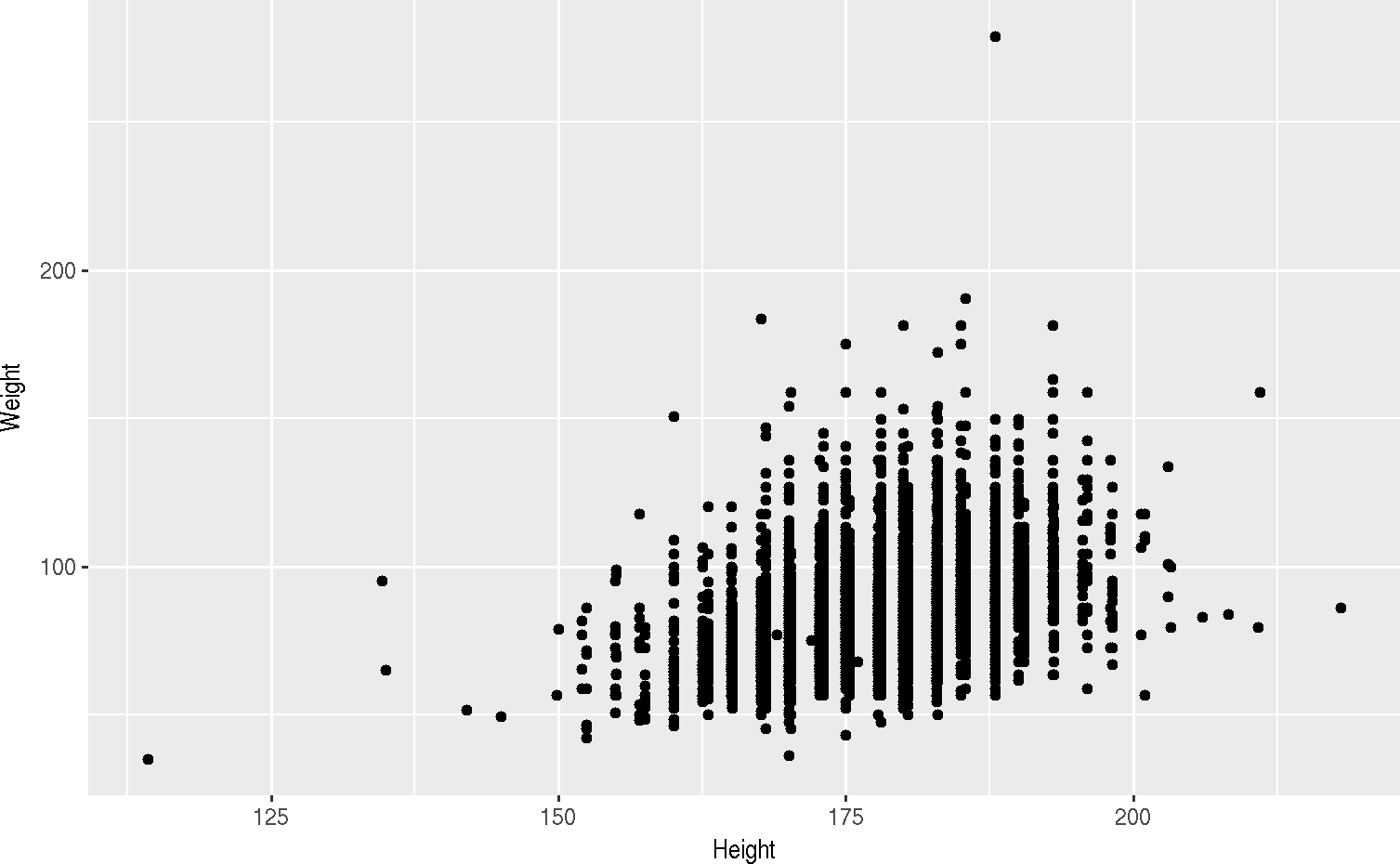Capture the base plot and points, and explore different smoothed relationships, e.g., linear model, non-parameteric smoother

plt <- ggplot(male, aes(x=Height, y = Weight)) + geom_point()
plt + geom_smooth(method = "lm")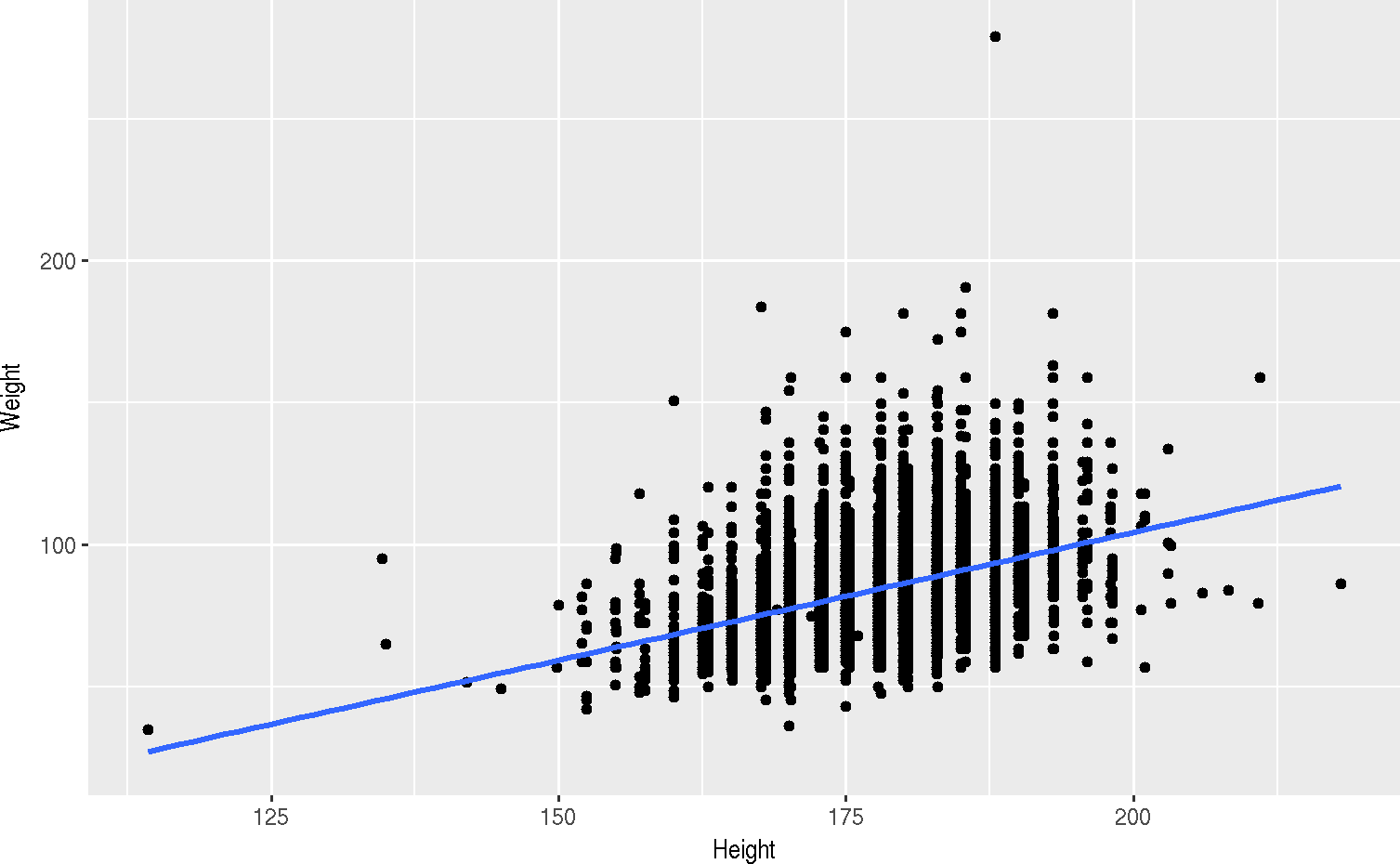plt + geom_smooth()       # default: generalized additive model
## geom_smooth() using method = 'gam' and formula 'y ~ s(x, bs = "cs")'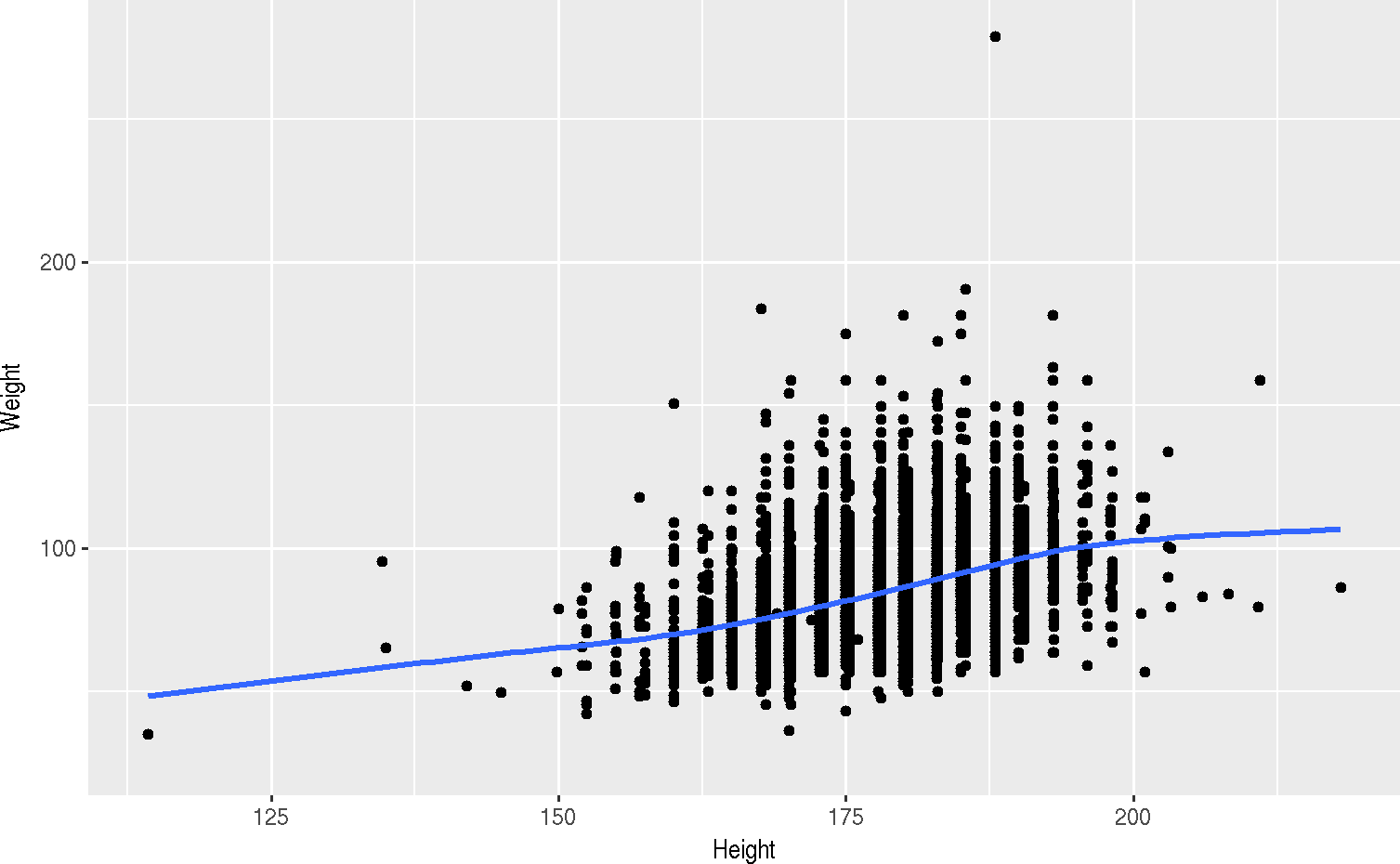Use an aes()thetic to color smoothed lines based on Year, or facet_grid() to separate years.

ggplot(male, aes(x = Weight)) + geom_density(aes(fill = Year), alpha = .2)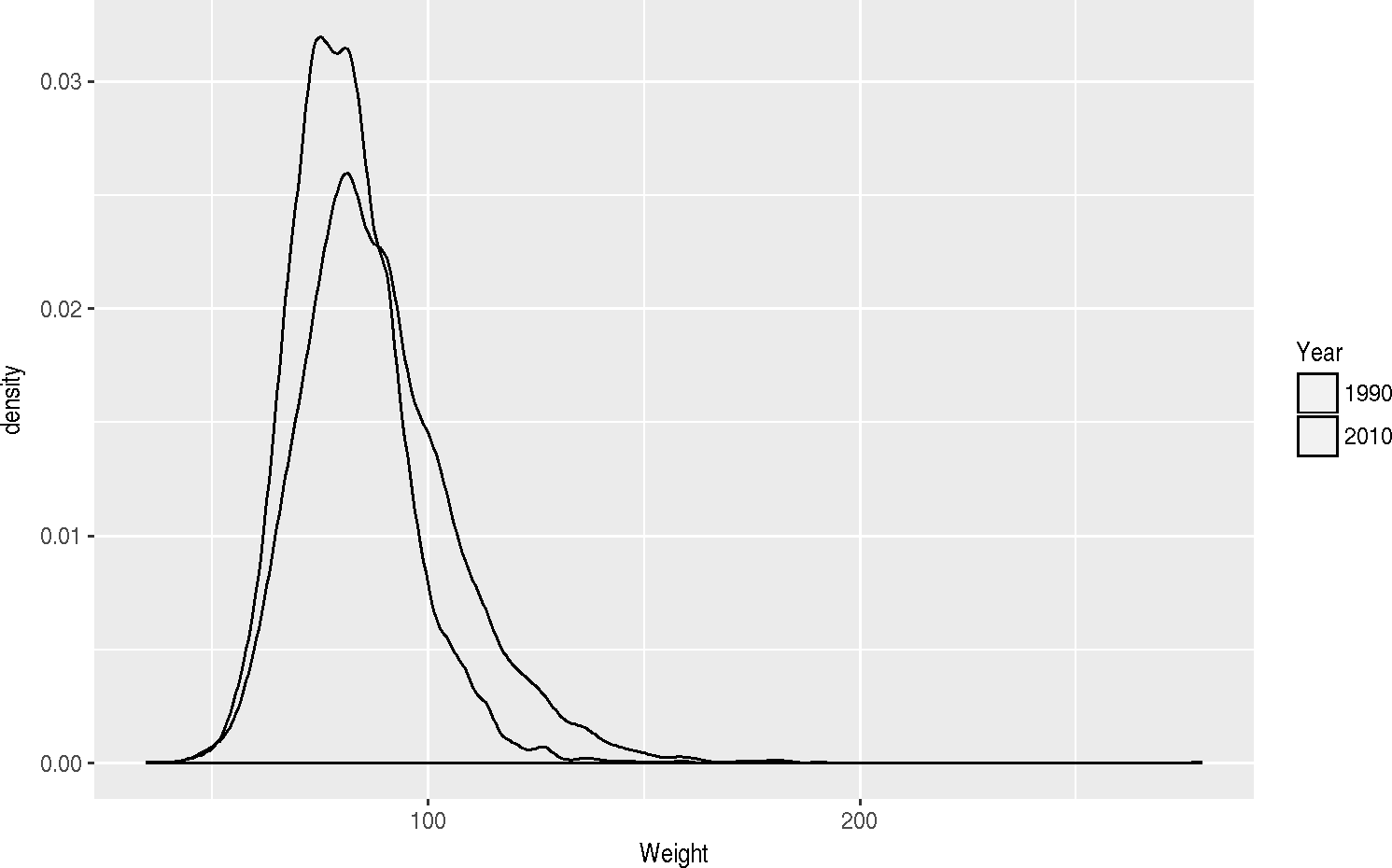plt + geom_smooth(method = "lm", aes(color = Year))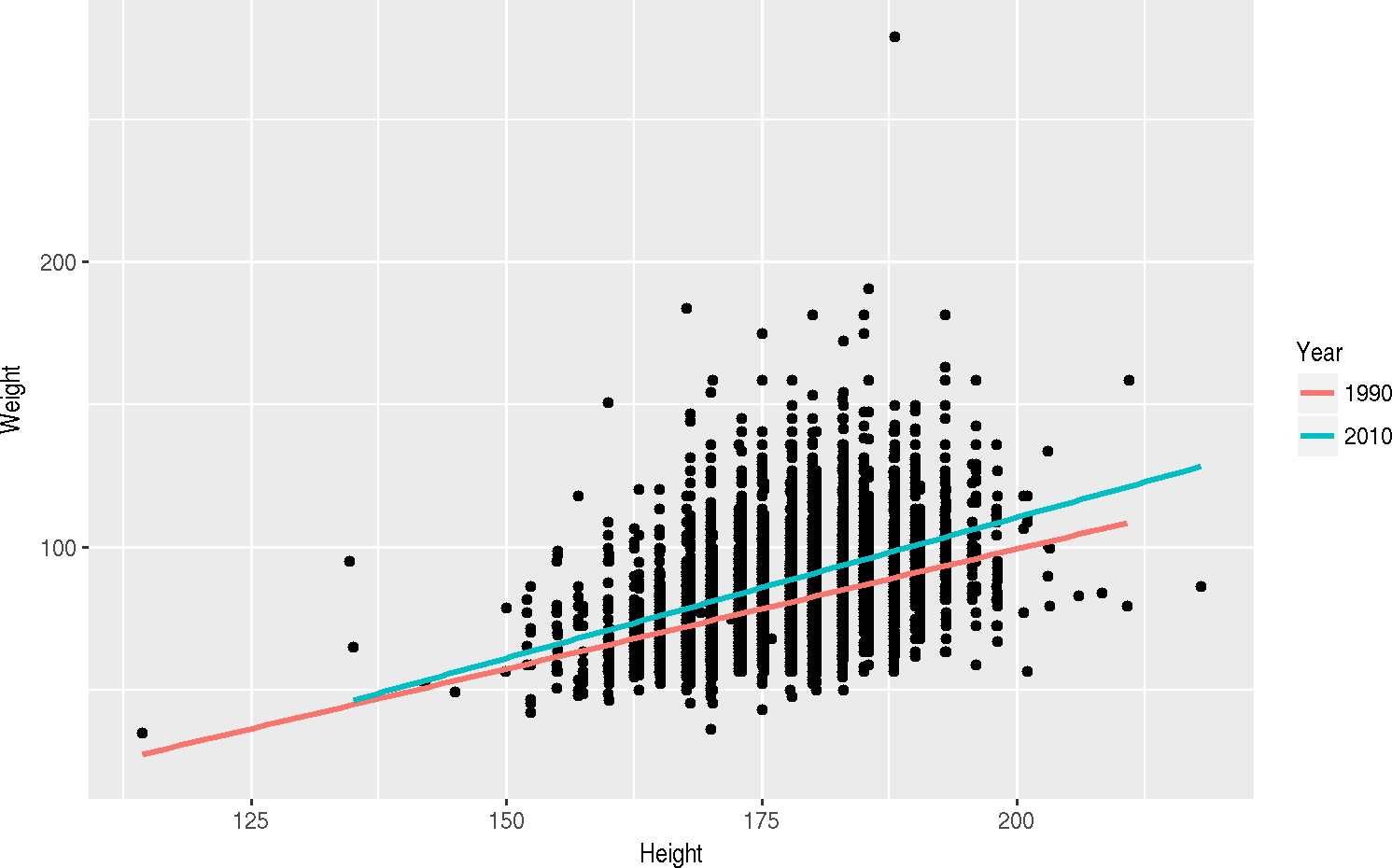plt + facet_grid( ~ Year ) + geom_smooth(method = "lm")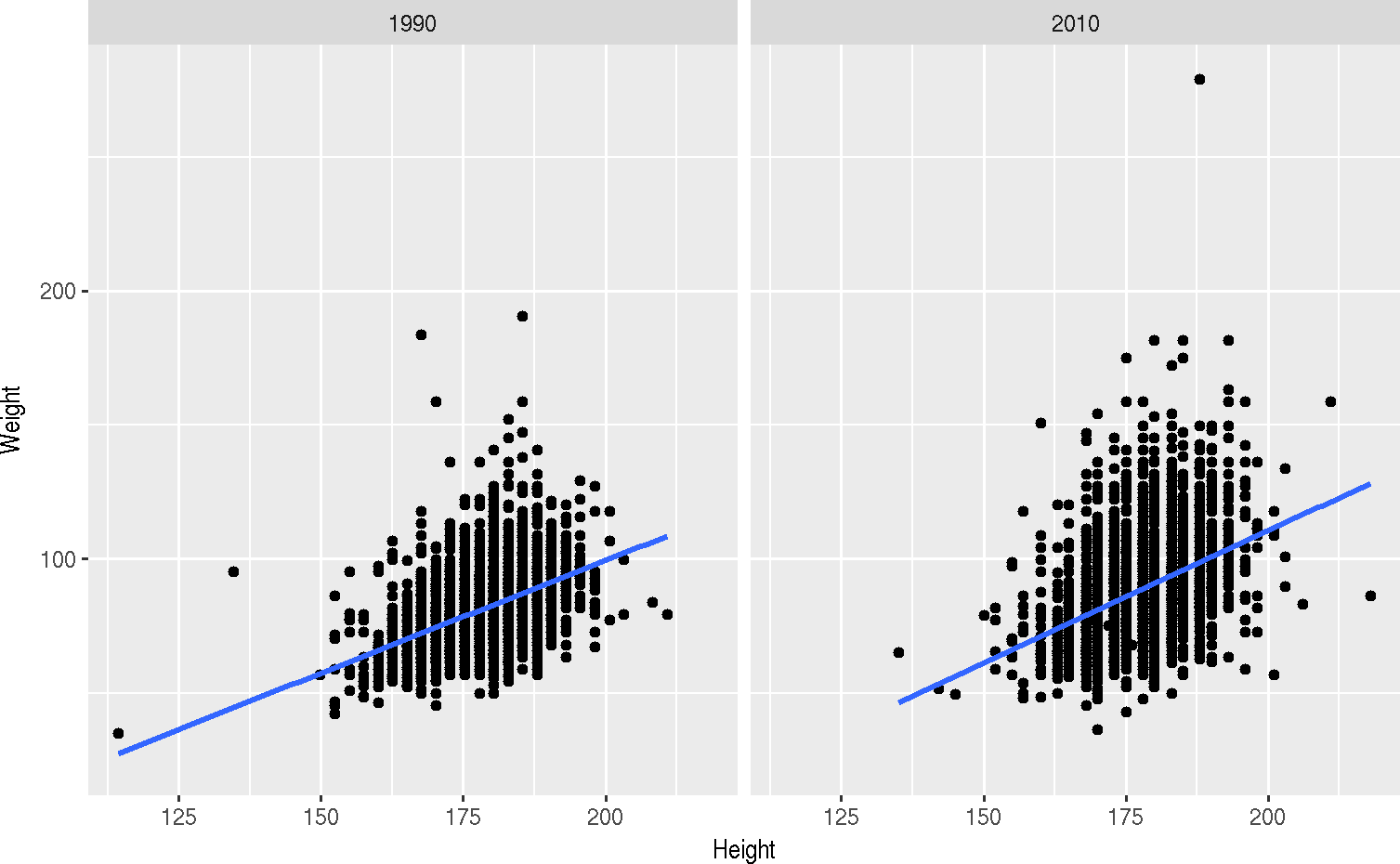# 2 Multivariate analysis

This is a classic microarray experiment. Microarrays consist of ‘probesets’ that interogate genes for their level of expression. In the experiment we’re looking at, there are 12625 probesets measured on each of the 128 samples. The raw expression levels estimated by microarray assays require considerable pre-processing, the data we’ll work with has been pre-processed.

## 2.1 Input and setup

Start by finding the expression data file on disk.

path <- file.choose()          # look for ALL-expression.csv
stopifnot(file.exists(path))

The data is stored in ‘comma-separate value’ format, with each probeset occupying a line, and the expression value for each sample in that probeset separated by a comma. Input the data using read_csv(). The sample identifiers are present in the first column.

exprs <- read_csv(path)
## Parsed with column specification:
## cols(
##   .default = col_double(),
##   Gene = col_character()
## )
## See spec(...) for full column specifications.

We’ll also input the data that describes each column

path <- file.choose()         # look for ALL-phenoData.csv
stopifnot(file.exists(path))
pdata <- read_csv(path)
## Parsed with column specification:
## cols(
##   .default = col_character(),
##   age = col_integer(),
##   t(4;11) = col_logical(),
##   t(9;22) = col_logical(),
##   cyto.normal = col_logical(),
##   ccr = col_logical(),
##   relapse = col_logical(),
##   transplant = col_logical()
## )
## See spec(...) for full column specifications.
pdata
## # A tibble: 128 x 22
##    Sample   cod  diagnosis   sex   age    BT remission    CR   date.cr
##     <chr> <chr>      <chr> <chr> <int> <chr>     <chr> <chr>     <chr>
##  1  01005  1005  5/21/1997     M    53    B2        CR    CR  8/6/1997
##  2  01010  1010  3/29/2000     M    19    B2        CR    CR 6/27/2000
##  3  03002  3002  6/24/1998     F    52    B4        CR    CR 8/17/1998
##  4  04006  4006  7/17/1997     M    38    B1        CR    CR  9/8/1997
##  5  04007  4007  7/22/1997     M    57    B2        CR    CR 9/17/1997
##  6  04008  4008  7/30/1997     M    17    B1        CR    CR 9/27/1997
##  7  04010  4010 10/30/1997     F    18    B1        CR    CR  1/7/1998
##  8  04016  4016  2/10/2000     M    16    B1        CR    CR 4/17/2000
##  9  06002  6002  3/19/1997     M    15    B2        CR    CR  6/9/1997
## 10  08001  8001  1/15/1997     M    40    B2        CR    CR 3/26/1997
## # ... with 118 more rows, and 13 more variables: t(4;11) <lgl>,
## #   t(9;22) <lgl>, cyto.normal <lgl>, citog <chr>, mol.biol <chr>, fusion
## #   protein <chr>, mdr <chr>, kinet <chr>, ccr <lgl>, relapse <lgl>,
## #   transplant <lgl>, f.u <chr>, date last seen <chr>

## 2.2 Cleaning and Exploration

The expression data is presented in what is sometimes called ‘wide’ format; a different format is ‘tall’, where Sample and Gene group the single observation Expression. Use tidyr::gather() to gather the columns of the wide format into two columns representing the tall format, excluding the Gene column from the gather operation.

exprs <- exprs %>% gather("Sample", "Expression", -Gene)

Explore the data a little, e.g., a summary and histogram of the expression values, and a histogram of average expression values of each gene.

exprs %>% select(Expression) %>% summary()
##    Expression
##  Min.   : 1.985
##  1st Qu.: 4.130
##  Median : 5.469
##  Mean   : 5.625
##  3rd Qu.: 6.827
##  Max.   :14.127
exprs $Expression %>% hist()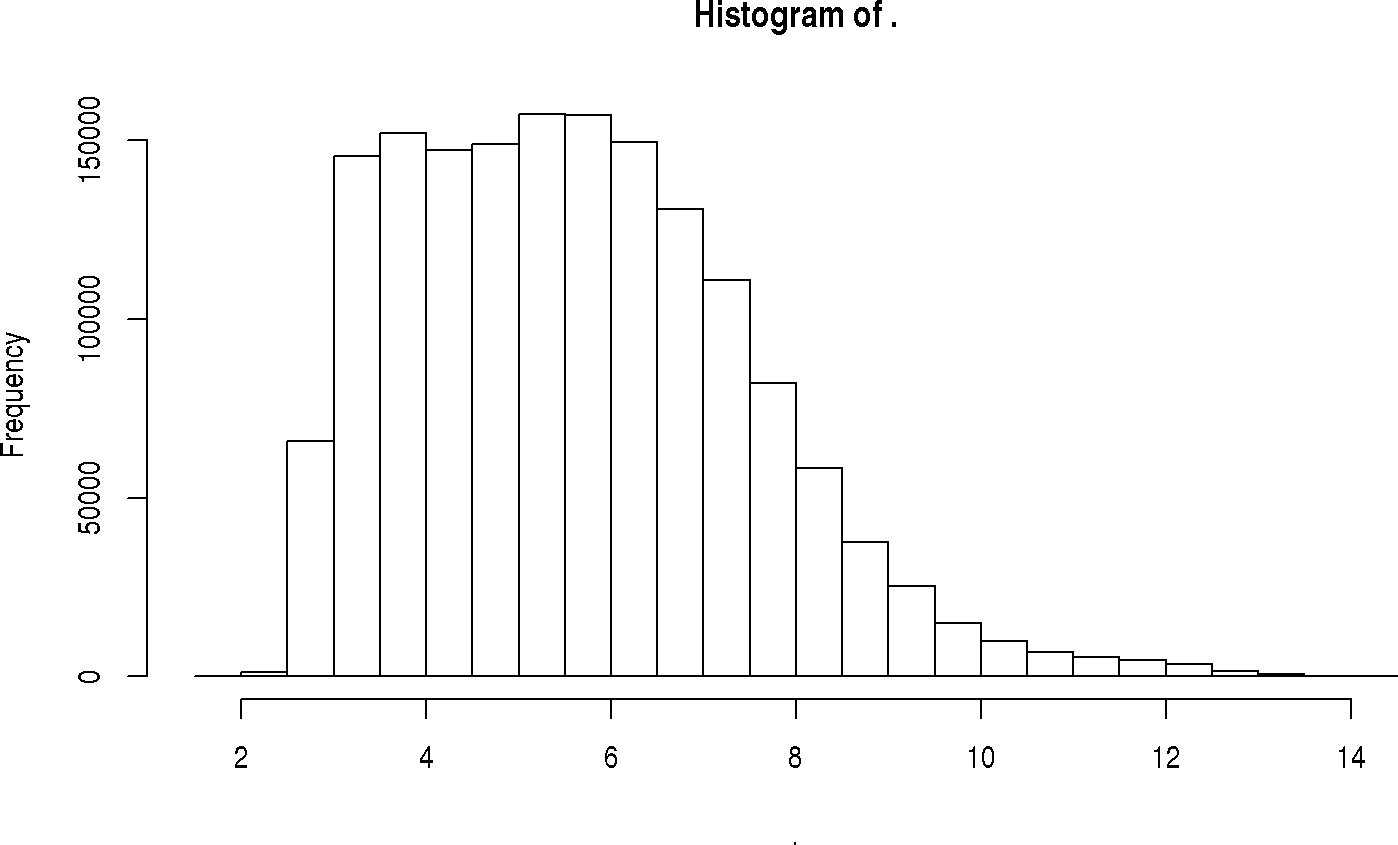exprs %>% group_by(Gene) %>% summarize(AveExprs = mean(Expression)) %$% AveExprs %>%
hist(breaks=50)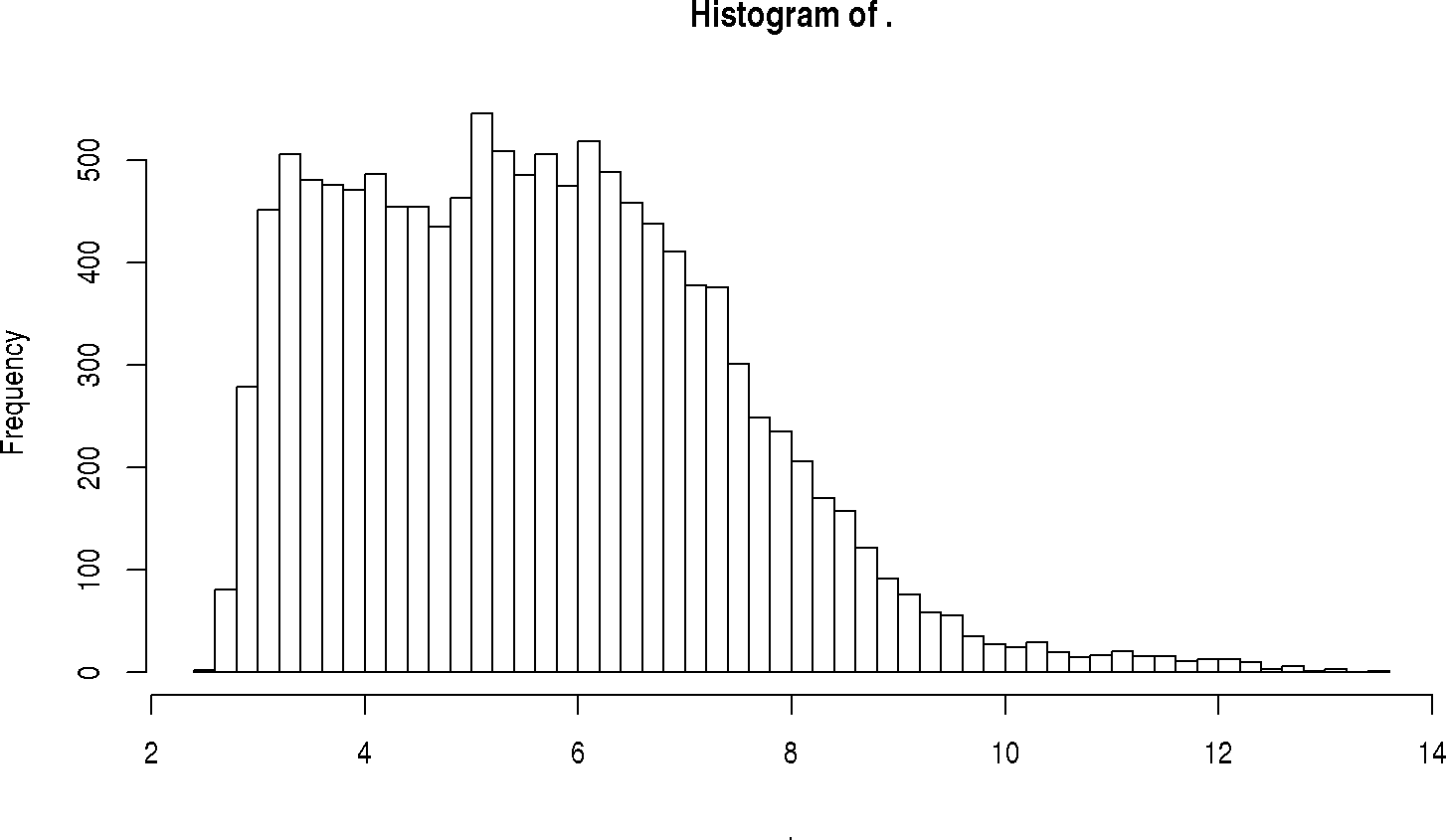For subsequent analysis, we also want to simplify the ‘B or T’ cell type classification

pdata <- pdata %>% mutate(B_or_T = factor(substr(BT, 1, 1)))

## 2.3 Unsupervised machine learning – multi-dimensional scaling

We’d like to reduce high-dimensional data to lower dimension for visualization. To do so, we need the dist()ance between samples. From ?dist, the input can be a data.frame where rows represent Sample and columns represent Expression values. Use spread() to create appropriate data from exprs, and pipe the result to dist()ance.x

input <- exprs %>% spread(Gene, Expression)
samples <- input \$ Sample
input <- input %>% select(-Sample) %>% as.matrix
rownames(input) <- samples

Calculate distance between samples, and use that for MDS scaling

mds <- dist(input) %>% cmdscale()

The result is a matrix; make it ‘tidy’ by coercing to a tibble; add the Sample identifiers as a distinct column.

mds <- mds %>% as.tibble() %>% mutate(Sample = rownames(mds))

Visualize the result

ggplot(mds, aes(x=V1, y = V2)) + geom_point()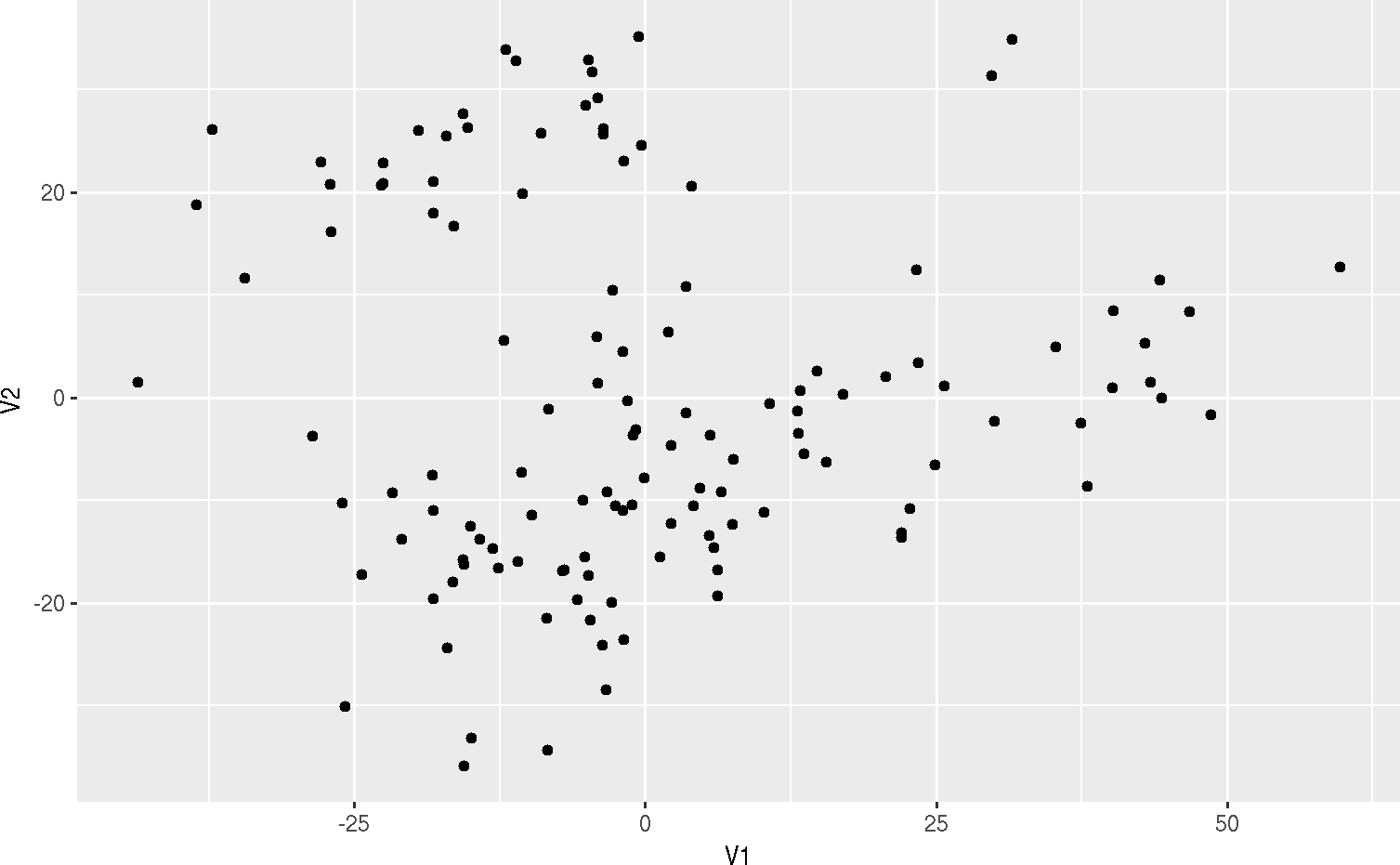With the ‘eye of faith’, it seems like there are two groups of points. To explore this, join the MDS scaling with the phenotypic data

joined <- inner_join(mds, pdata)
## Joining, by = "Sample"

and use the B_or_T column as an aesthetic to color points

ggplot(joined, aes(x = V1, y = V2)) + geom_point(aes(color = B_or_T))